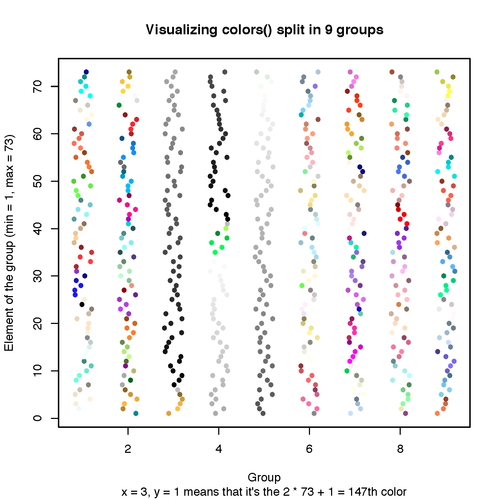Want to share your content on R-bloggers? click here if you have a blog, or here if you don't.

The other day I learnt about the existance of the colors() vector in R which specifies all the character-based colors like “light blue”, “black”, etc. So I made a simple plot to visualize them all. Here’s the code:

```mat <- matrix(1:length(colors()), ncol = 9, byrow= TRUE)
df <- data.frame(col = colors(),
x = as.integer(cut(1:length(colors()), 9)),
y = rep(1:73, 9), stringsAsFactors=FALSE)
plot(y ~ jitter(x), data = df, col = df\$col,
pch=16, main = "Visualizing colors() split in 9 groups",
xlab = "Group",
ylab = "Element of the group (min = 1, max = 73)",
sub = "x = 3, y = 1 means that it's the 2 * 73 + 1 = 147th color")```

And the plot:Hm… the code should look more pretty (see screenshot below), but Tumblr edits the html code. If you know how to deal with this, let me know.